# Interpret Deep Learning Time-Series Classifications Using Grad-CAM

This example shows how to use the gradient-weighted class activation mapping (Grad-CAM) technique to understand the classification decisions of a 1-D convolutional neural network trained on time-series data.

Grad-CAM  uses the gradient of the classification score with respect to the convolutional features determined by the network to understand which parts of the data are most important for classification. For time-series data, Grad-CAM computes the most important time steps for the classification decision of the network.

This image shows an example sequence with a Grad-CAM importance colormap. The map highlights the regions the network uses to make the classification decision.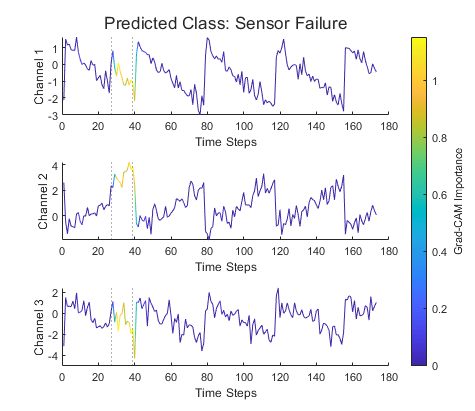This example uses supervised learning on labeled data to classify time-series data as "Normal" or "Sensor Failure". You can also use an autoencoder network to perform time-series anomaly detection on unlabeled data. For more information, see Time Series Anomaly Detection Using Deep Learning.

Load the Waveform data set from `WaveformData.mat`. This data set contains synthetically generated waveforms of varying length. Each waveform has three channels.

```rng("default") load WaveformData numChannels = size(data{1},1); numObservations = numel(data);```

Visualize the first few sequences in a plot.

```figure tiledlayout(2,2) for i = 1:4 nexttile stackedplot(data{i}',DisplayLabels="Channel "+(1:numChannels)); title("Observation "+i) xlabel("Time Step") end```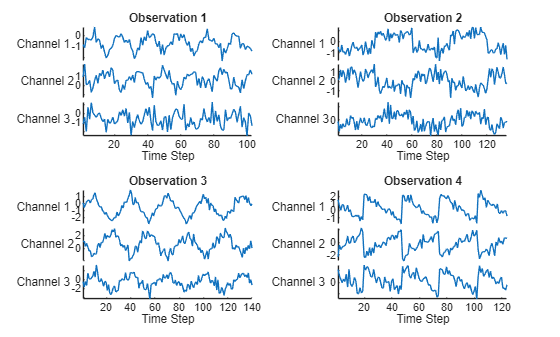### Simulate Sensor Failure

Create a new set of data by manually editing some of the sequences to simulate sensor failure.

Create a copy of the unmodified data.

`dataUnmodified = data;`

Randomly select 10% of the sequences to modify.

```failureFraction = 0.1; numFailures = round(numObservations*failureFraction); failureIdx = randperm(numel(data),numFailures);```

To simulate the sensor failure, introduce a small additive anomaly between 0.25 and 2 in height. Each anomaly occurs at a random place in the sequence and occurs for between four and 20 time steps.

```anomalyHeight = [0.25 2]; anomalyPatchSize = [4 20]; anomalyHeightRange = anomalyHeight(2) - anomalyHeight(1);```

Modify the sequences.

```failureLocation = cell(size(data)); for i = 1:numFailures X = data{failureIdx(i)}; % Generate sensor failure location. patchLength = randi(anomalyPatchSize,1); patchStart = randi(length(X)-patchLength); idxPatch = patchStart:(patchStart+patchLength); % Generate anomaly height. patchExtraHeight = anomalyHeight(1) + anomalyHeightRange*rand(1,1); X(:,idxPatch) = X(:,idxPatch) + patchExtraHeight; % Save modified sequence. data{failureIdx(i)} = X; % Save failure location. failureLocation{failureIdx(i)} = idxPatch; end```

For the unmodified sequences, set the class label to `Normal`. For the modified sequences, set the class label to `Sensor Failure`.

```labels = repmat("Normal",numObservations,1); labels(failureIdx) = "Sensor Failure"; labels = categorical(labels);```

Visualize the class label distribution using a histogram.

```figure histogram(labels)```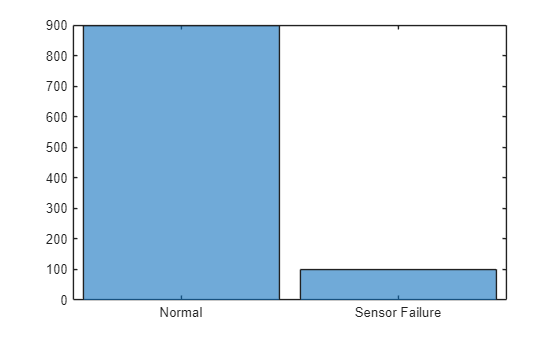#### Visualize Sensor Failures

Compare a selection of modified sequences with the original sequences. The dashed lines indicate the region of the sensor failure.

```numFailuresToShow = 2; for i=1:numFailuresToShow figure t = tiledlayout(numChannels,1); idx = failureIdx(i); modifiedSignal = data{idx}; originalSignal = dataUnmodified{idx}; for j = 1:numChannels nexttile plot(modifiedSignal(j,:)) hold on plot(originalSignal(j,:)) ylabel("Channel "+j) xlabel("Time Step") xline(failureLocation{idx}(1),":") xline(failureLocation{idx}(end),":") hold off end title(t,"Observation "+failureIdx(i)) legend("Modified","Original", ... Location="southoutside", ... NumColumns=2) end```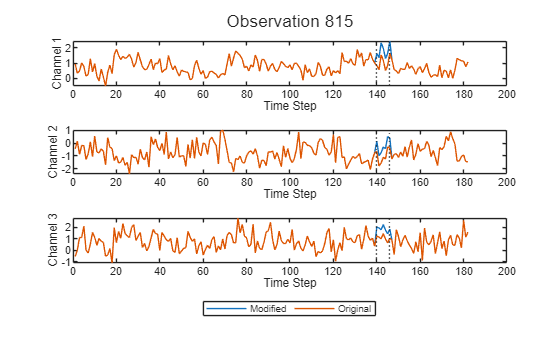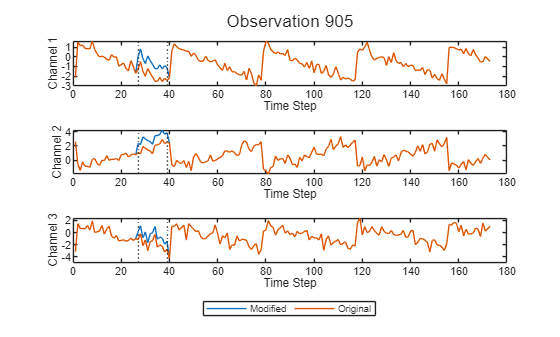The modified and original signals match except for the anomalous patch corresponding to the sensor failure.

### Prepare Data

Prepare the data for training by splitting the data into training and validation sets. Use 90% of the data for training and 10% of the data for validation.

```trainFraction = 0.9; idxTrain = 1:floor(trainFraction*numObservations); idxValidation = (idxTrain(end)+1):numObservations; XTrain = data(idxTrain); TTrain = labels(idxTrain); XValidation = data(idxValidation); TValidation = labels(idxValidation); failureLocationValidation = failureLocation(idxValidation);```

### Define Network Architecture

Define the 1-D convolutional neural network architecture.

• Use a sequence input layer with an input size that matches the number of channels of the input data.

• Specify two blocks of 1-D convolution, ReLU, and layer normalization layers, where the convolutional layer has a filter size of 3. Specify 32 and 64 filters for the first and second convolutional layers, respectively. For both convolutional layers, left-pad the inputs such that the outputs have the same length (causal padding).

• To reduce the output of the convolutional layers to a single vector, use a 1-D global average pooling layer.

• To map the output to a vector of probabilities, specify a fully connected layer with an output size matching the number of classes, followed by a softmax layer and a classification layer.

```classes = categories(TTrain); numClasses = numel(classes); filterSize = 3; numFilters = 32; layers = [ ... sequenceInputLayer(numChannels) convolution1dLayer(filterSize,numFilters,Padding="causal") reluLayer layerNormalizationLayer(OperationDimension="batch-excluded") convolution1dLayer(filterSize,2*numFilters,Padding="causal") reluLayer layerNormalizationLayer(OperationDimension="batch-excluded") globalAveragePooling1dLayer fullyConnectedLayer(numClasses) softmaxLayer classificationLayer];```

### Specify Training Options

Train the network using adaptive momentum (ADAM). Set the maximum number of epochs to 15 and use a mini-batch size of 27. Left-pad all the sequences in a mini-batch to be the same length. Use validation data to validate the network during training. Monitor the training progress in a plot and suppress the verbose output.

```miniBatchSize = 27; options = trainingOptions("adam", ... MiniBatchSize=miniBatchSize, ... MaxEpochs=15, ... SequencePaddingDirection="left", ... ValidationData={XValidation,TValidation}, ... Plots="training-progress", ... Verbose=false);```

### Train Network

Train the convolutional network with the specified options using the `trainNetwork` function.

`net = trainNetwork(XTrain,TTrain,layers,options);`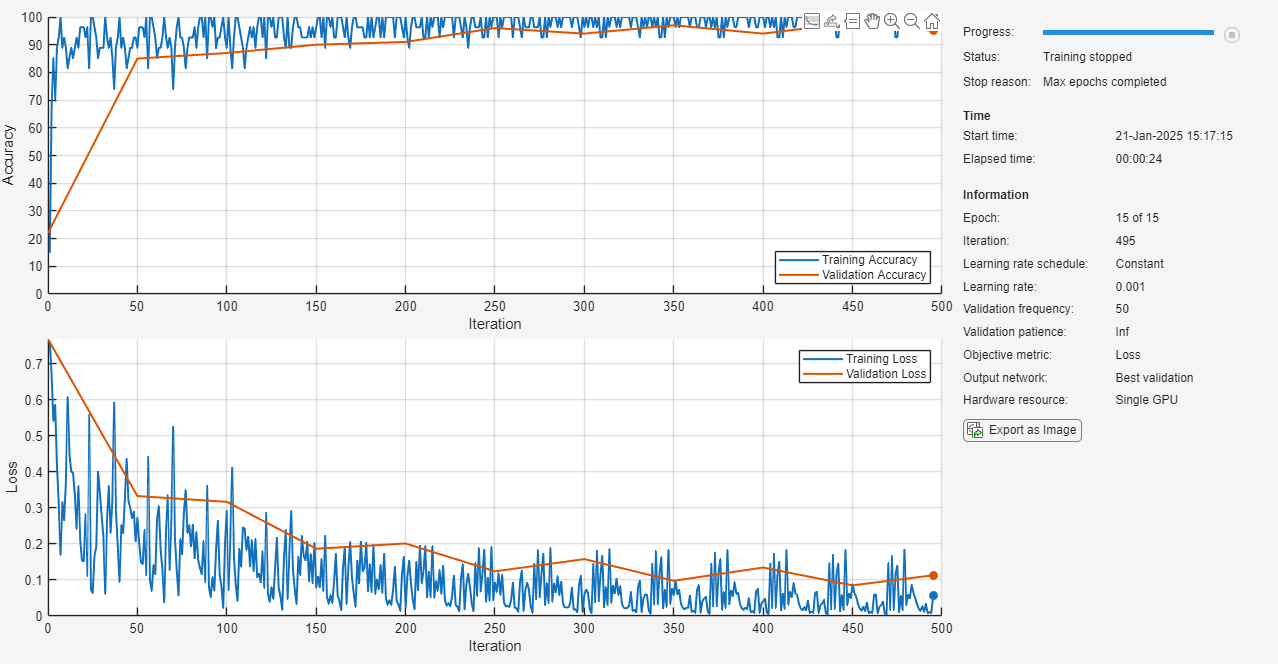### Test Network

Classify the validation data using the same mini-batch size and sequence padding options used for training.

```YValidation = classify(net,XValidation, ... MiniBatchSize=miniBatchSize, ... SequencePaddingDirection="left");```

Calculate the classification accuracy of the predictions.

`accuracy = mean(YValidation == TValidation)`
```accuracy = 0.9500 ```

Visualize the predictions in a confusion matrix.

```figure confusionchart(TValidation,YValidation)```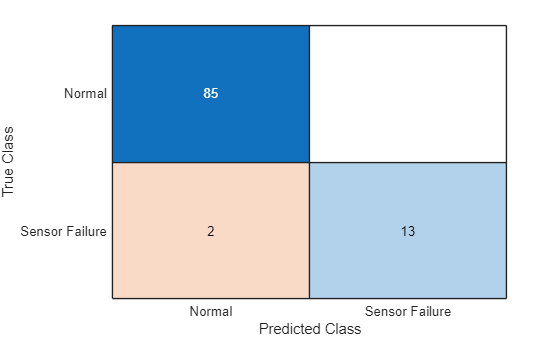### Use Grad-CAM to Interpret Classification Results

Use Grad-CAM to visualize the parts of the sequence that the network uses to make the classification decisions.

Find a subset of sequences that the network correctly classifies as "Sensor Failure".

```numFailuresToShow = 2; isCorrect = TValidation == "Sensor Failure" & YValidation == "Sensor Failure"; idxValidationFailure = find(isCorrect,numFailuresToShow);```

For each observation, compute and visualize the Grad-CAM map. To compute the Grad-CAM importance map, use `gradCAM`. Display a colormap representing the Grad-CAM importance using the `plotWithColorGradient` helper function, defined at the end of this example. Add dashed lines to show the true location of the sensor failure.

```for i = 1:numFailuresToShow figure t = tiledlayout(numChannels,1); idx = idxValidationFailure(i); modifiedSignal = XValidation{idx}; importance = gradCAM(net,modifiedSignal,"Sensor Failure"); for j = 1:numChannels nexttile plotWithColorGradient(modifiedSignal(j,:)',importance'); ylabel("Channel "+j) xlabel("Time Steps") if ~isempty(failureLocationValidation{idx}) xline(failureLocationValidation{idx}(1),":") xline(failureLocationValidation{idx}(end),":") end end title(t,"Grad-CAM: Validation Observation "+idx) c = colorbar; c.Layout.Tile = "east"; c.Label.String = "Grad-CAM Importance"; end```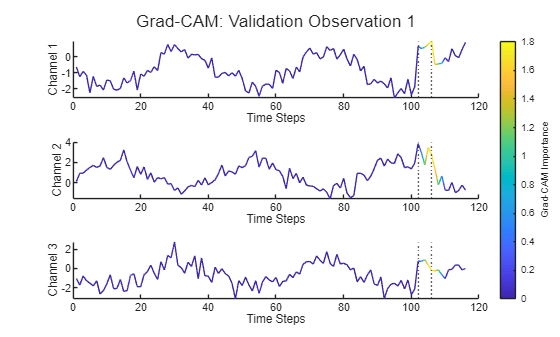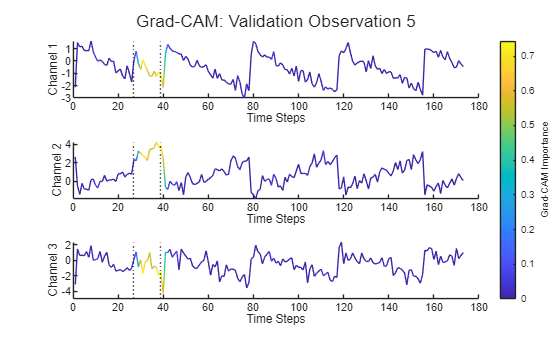The Grad-CAM map shows that the network is correctly using the sensor failure regions of the sequence to make the classification decisions. Use of the correct regions suggests that the network is learning how to discriminate between normal and failing data. The network is using the failure to decide, rather than spurious background features.

### Use Grad-CAM to Investigate Misclassifications

You can also use Grad-CAM to investigate misclassified sequences.

Find a subset of sensor failure sequences that the network misclassifies as "Normal".

```numFailuresToShow = 2; isIncorrect = TValidation == "Sensor Failure" & YValidation == "Normal"; idxValidationFailure = find(isIncorrect,numFailuresToShow);```

For each misclassification, compute and visualize the Grad-CAM map. For the misclassified sensor failure sequences, the Grad-CAM map shows that the network does find the failure region. However, unlike the correctly classified sequences, the network does not use the entire failure region to make the classification decision.

```for i = 1:length(idxValidationFailure) figure t = tiledlayout(numChannels,1); idx = idxValidationFailure(i); modifiedSignal = XValidation{idx}; importance = gradCAM(net,modifiedSignal,"Sensor Failure"); for j = 1:numChannels nexttile plotWithColorGradient(modifiedSignal(j,:)',importance'); ylabel("Channel "+j) xlabel("Time Steps") if ~isempty(failureLocationValidation{idx}) xline(failureLocationValidation{idx}(1),":") xline(failureLocationValidation{idx}(end),":") end end title(t,"Grad-CAM: Validation Observation "+idx) c = colorbar; c.Layout.Tile = "east"; c.Label.String = "Grad-CAM Importance"; end```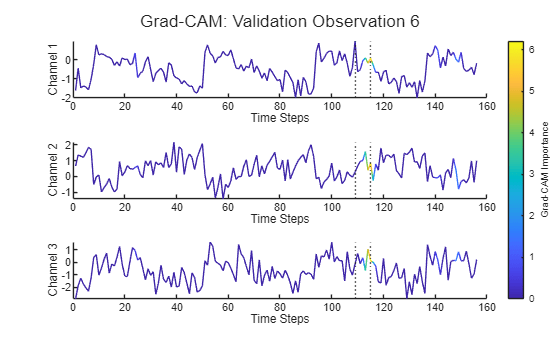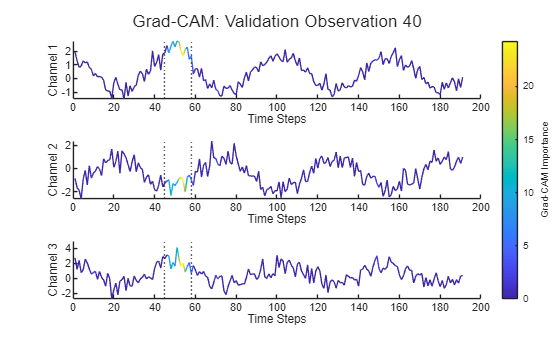### Helper Function

The `plotWithColorGradient` function takes as input a sequence with a single channel and an importance map with the same number of time steps as the sequence. The function uses the importance map to color segments of the sequence.

Set the last entry of `y` and `c` to `NaN` so that patch creates a line instead of a closed polygon.

```function plotWithColorGradient(sequence,importance) x = 1:size(sequence,1) + 1; y = [sequence; NaN]; c = [importance; NaN]; patch(x,y,c,EdgeColor="interp"); end```

 Selvaraju, Ramprasaath R., Michael Cogswell, Abhishek Das, Ramakrishna Vedantam, Devi Parikh, and Dhruv Batra. “Grad-CAM: Visual Explanations from Deep Networks via Gradient-Based Localization.” International Journal of Computer Vision 128, no. 2 (February 2020): 336–59. https://doi.org/10.1007/s11263-019-01228-7.